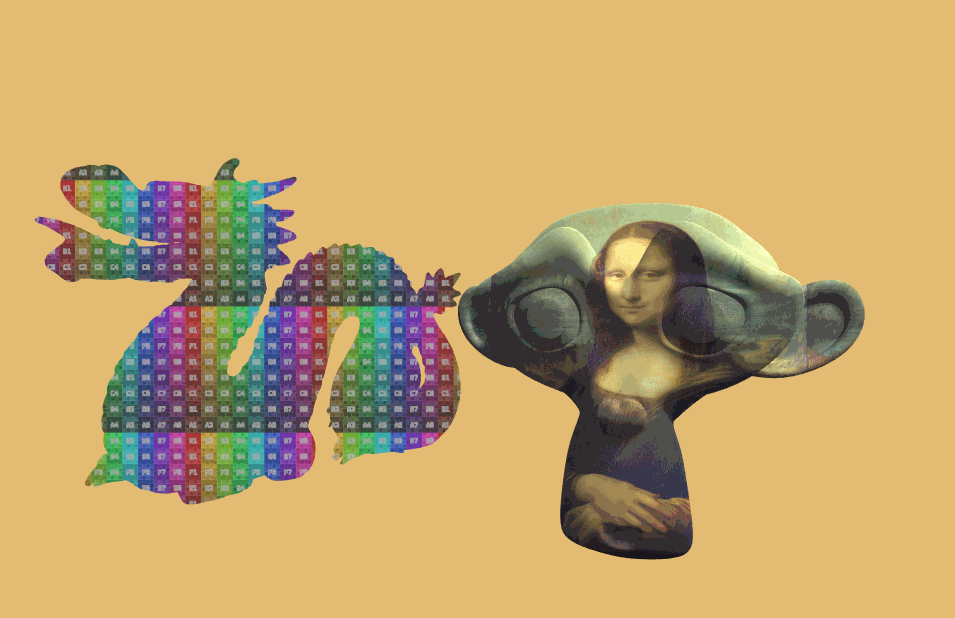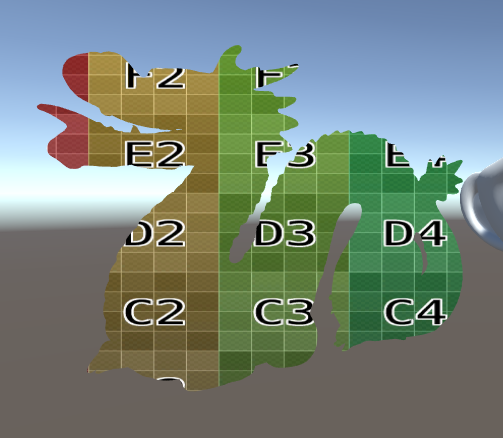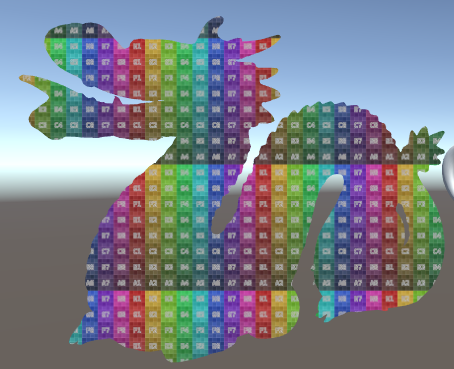There are many techniques how to generate texture coordinates. Previous tutorials explain how to use UV coordinates and how to generate coordinates based on planar and triplanar mapping. In this one we’ll use the position of the pixel on the screen as the coordinate.

On it’s own the effect just looks kind of weird, which can also be used as a aesthetic choice, but it can be used for many cool effects I’ll go into in the future.If you’re new to shader programming I recommend you to read my tutorial on the basics of writing shaders first. If you’ve written a few shaders you should be fine. As the base shader I’ll iterate upon I’ll use the result of the tutorial about using textures in a shader.

The first changes we make is to expand the vertex to fragment struct. So far it held the position and the uv coordinates. The screen position is used similarly to the uv coordinates, but for the screen position we need a vector with 4 components instead of 2.

``````//the data that's used to generate fragments and can be read by the fragment shader
struct v2f{
float4 position : SV_POSITION;
float4 screenPosition : TEXCOORD0;
};
``````

Then we fill that new variable in the vertex function. We can get the screen position from the clip space position via a function in the unity shader library called `ComputeScreenPos`. We simply pass it the position in clipspace (the result of the UnityObjectToClipPos function) and it’ll return the screenspace position.

``````//the vertex shader
v2f vert(appdata v){
v2f o;
//convert the vertex positions from object space to clip space so they can be rendered
o.position = UnityObjectToClipPos(v.vertex);
o.screenPosition = ComputeScreenPos(o.position);
return o;
}
``````

In the fragment shader we take the first two components of the screen position and divide them by the last one. This division is to counteract the perspective correction the GPU automatically performs on interpolators.

``````//the fragment shader
fixed4 frag(v2f i) : SV_TARGET{
float2 textureCoordinate = i.screenPosition.xy / i.screenPosition.w;
fixed4 col = tex2D(_MainTex, textureCoordinate);
col *= _Color;
return col;
}
``````This already works as expected, but when our screen isn’t square(like it usually isn’t) our image will be distorted, additionally the screen position doesn’t take the scaling and translation of the texture into consideration we set in the inspector.

To fix the stretching we can simply multiply the x component of the coordinate by the aspect ratio. We get the aspect ratio by dividing the width of the current rendertarget by it’s height. Those variables are saved in the x and y component of the `_ScreenParams` variable.

``````//the fragment shader
fixed4 frag(v2f i) : SV_TARGET{
float2 textureCoordinate = i.screenPosition.xy / i.screenPosition.w;
float aspect = _ScreenParams.x / _ScreenParams.y;
textureCoordinate.x = textureCoordinate.x * aspect;
fixed4 col = tex2D(_MainTex, textureCoordinate);
col *= _Color;
return col;
}
``````

To apply the scaling and offset of the texture we can then use the TRANSFORM_TEX macro which we previously used in the vertex shader.

``````//the fragment shader
fixed4 frag(v2f i) : SV_TARGET{
float2 textureCoordinate = i.screenPosition.xy / i.screenPosition.w;
float aspect = _ScreenParams.x / _ScreenParams.y;
textureCoordinate.x = textureCoordinate.x * aspect;
textureCoordinate = TRANSFORM_TEX(textureCoordinate, _MainTex);
fixed4 col = tex2D(_MainTex, textureCoordinate);
col *= _Color;
return col;
}
``````To use screenspace coordinates in surface shaders I recommend you to learn how surface shaders in general work, I have a tutorial about that here. I’ll also use the result of that tutorial as a starting point for this shader.

For surface shaders we don’t have to prepare anything in the vertex shader, just adding a variable called `screenPos` to the surface input struct will make unity generate code that fills it with the correct data.

``````struct Input {
float4 screenPos;
};
``````

We can then use this variable the same way we used it in the unlit shader. We just have to add the `_MainTex_ST` variable to the uniform variables because unity won’t generate that unless we have a variable called `uv_MainTex` in our input struct.

``````//uniform variables
float4 _MainTex_ST;
``````
``````void surf (Input i, inout SurfaceOutputStandard o) {
float2 textureCoordinate = i.screenPos.xy / i.screenPos.w;
float aspect = _ScreenParams.x / _ScreenParams.y;
textureCoordinate.x = textureCoordinate.x * aspect;
textureCoordinate = TRANSFORM_TEX(textureCoordinate, _MainTex);

fixed4 col = tex2D(_MainTex, textureCoordinate);
col *= _Color;
o.Albedo = col.rgb;
o.Metallic = _Metallic;
o.Smoothness = _Smoothness;
o.Emission = _Emission;
}
``````Source

Unlit Screenspace Texture

``````Shader "Tutorial/039_ScreenspaceTextures/Unlit"{
//show values to edit in inspector
Properties{
_Color ("Tint", Color) = (0, 0, 0, 1)
_MainTex ("Texture", 2D) = "white" {}
}

//the material is completely non-transparent and is rendered at the same time as the other opaque geometry
Tags{ "RenderType"="Opaque" "Queue"="Geometry"}

Pass{
CGPROGRAM

#include "UnityCG.cginc"

#pragma vertex vert
#pragma fragment frag

//texture and transforms of the texture
sampler2D _MainTex;
float4 _MainTex_ST;

//tint of the texture
fixed4 _Color;

//the object data that's put into the vertex shader
struct appdata{
float4 vertex : POSITION;
};

//the data that's used to generate fragments and can be read by the fragment shader
struct v2f{
float4 position : SV_POSITION;
float4 screenPosition : TEXCOORD0;
};

v2f vert(appdata v){
v2f o;
//convert the vertex positions from object space to clip space so they can be rendered
o.position = UnityObjectToClipPos(v.vertex);
o.screenPosition = ComputeScreenPos(o.position);
return o;
}

fixed4 frag(v2f i) : SV_TARGET{
float2 textureCoordinate = i.screenPosition.xy / i.screenPosition.w;
float aspect = _ScreenParams.x / _ScreenParams.y;
textureCoordinate.x = textureCoordinate.x * aspect;
textureCoordinate = TRANSFORM_TEX(textureCoordinate, _MainTex);
fixed4 col = tex2D(_MainTex, textureCoordinate);
col *= _Color;
return col;
}

ENDCG
}
}
}
``````

Lit Screenspace Texture

``````Shader "Tutorial/039_ScreenspaceTextures/Surface" {
Properties {
_Color ("Tint", Color) = (0, 0, 0, 1)
_MainTex ("Texture", 2D) = "white" {}
_Smoothness ("Smoothness", Range(0, 1)) = 0
_Metallic ("Metalness", Range(0, 1)) = 0
[HDR] _Emission ("Emission", color) = (0,0,0)
}
Tags{ "RenderType"="Opaque" "Queue"="Geometry"}

CGPROGRAM

#pragma target 3.0

sampler2D _MainTex;
float4 _MainTex_ST;
fixed4 _Color;

half _Smoothness;
half _Metallic;
half3 _Emission;

struct Input {
float4 screenPos;
};

void surf (Input i, inout SurfaceOutputStandard o) {
float2 textureCoordinate = i.screenPos.xy / i.screenPos.w;
float aspect = _ScreenParams.x / _ScreenParams.y;
textureCoordinate.x = textureCoordinate.x * aspect;
textureCoordinate = TRANSFORM_TEX(textureCoordinate, _MainTex);

fixed4 col = tex2D(_MainTex, textureCoordinate);
col *= _Color;
o.Albedo = col.rgb;
o.Metallic = _Metallic;
o.Smoothness = _Smoothness;
o.Emission = _Emission;
}
ENDCG
}
FallBack "Standard"
}
``````

You can also find me on twitter at @totallyRonja. If you liked my tutorial and want to support me you can do that on Patreon (patreon.com/RonjaTutorials) or Ko-Fi (ko-fi.com/RonjaTutorials).